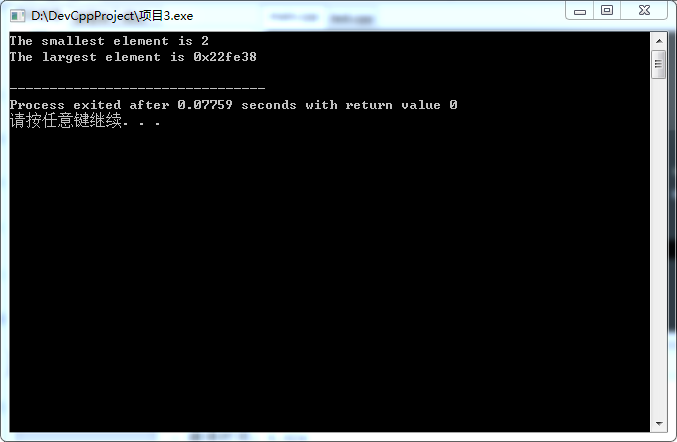• ## c++max函数小坑

千次阅读 2019-07-18 09:22:00
今天做牛客网的在线编程题，有道题用到了max函数，发现了一个小坑，比如$$max(a,0)$$时，若a是long long 类型，就会报错，需要将0改成0ll. 转载于:https://www.cnblogs.com/yy-moon/p/11205057.html...
今天做牛客网的在线编程题，有道题用到了max函数，发现了一个小坑，比如$$max(a,0)$$时，若a是long long 类型，就会报错，需要将0改成0ll.

转载于:https://www.cnblogs.com/yy-moon/p/11205057.html
展开全文• C++中，根据函数能否被其他源文件调用，将函数区分为内部函数和外部函数。 内部函数是指一个函数只能被本文件中其他函数所调用，在定义内部函数时，在函数名和函数类型的前面加static。 函数首部的一般格式为 ...
C++内部函数
在C++中，根据函数能否被其他源文件调用，将函数区分为内部函数和外部函数。
内部函数是指一个函数只能被本文件中其他函数所调用，在定义内部函数时，在函数名和函数类型的前面加static。
函数首部的一般格式为
static 类型标识符 函数名（形参表）
//如
static int Max(int num1,int num2)

内部函数又称静态函数。在C++中使用内部函数，可以使函数只局限于所在文件。如果在不同的文件中有同名的内部函数，互不干扰。
C++外部函数
在C++中定义函数时，如果在函数首部的最左端加上关键字extern，则表示此函数是外部函数，可供其他文件调用。
//如函数开头写
extern int Max(int num1,int num2)

按照上述定义，函数Max就可以为其他文件调用。如果在定义函数时省略extern，则默认为外部函数。
经典案例：C++实现调用外部Max函数。
源码分析：
第一步：单独创建一个Max.app的文件，编写代码：
#include<iostream>//头文件
using namespace std;//命名空间
int Max(int x,int y)//自定义Max函数
{
int temp;//定义中间变量
temp=x>y?x:y;//把x y中的较大值赋给temp
return temp;//函数返回值为temp
}

第二步：在头文件添加Max函数，此文件保存格式为.h：
#include<iostream>//头文件
int Max(int x,int y);

第三步：编写主函数内容：
#include<iostream>//头文件
#include "lx.h"//注意此处
using namespace std;//命名空间
int main()//主函数
{
extern int Max(int x,int y);//声明外部函数
int num1,num2,maxNumber;//定义变量
cin>>num1>>num2;//输入两个数
maxNumber=max(num1,num2);//把较大的赋值给maxNumber
cout<<maxNumber;//输出maxNumber
return 0;//函数返回值为0
}

编译后的结果：
44 90
90
--------------------------------
Process exited after 5.853 seconds with return value 0
请按任意键继续. . .

C++调用外部Max函数
更多案例可以go公众号：C语言入门到精通


展开全文编程语言
• c++minmax函数 C ++ STL std :: minmax()函数 (C++ STL std::minmax() function) minmax() function is a library function of algorithm header, it is used to find the smallest and largest values, it accepts ...
c++minmax函数 C ++ STL std :: minmax()函数 (C++ STL std::minmax() function)
minmax() function is a library function of algorithm header, it is used to find the smallest and largest values, it accepts two values and returns a pair of the smallest and largest values, the first element of the pair contains the smallest value and the second element of the pair contains the largest value.
minmax()函数是算法标头的库函数，用于查找最小和最大值，它接受两个值并返回一对最小和最大值，该对中的第一个元素包含最小值，并且该对中的第二个元素包含最大值。
Note:To use minmax() function – include <algorithm> header or you can simple use <bits/stdc++.h> header file.
注意：要使用minmax()函数 –包括<algorithm>头文件，或者您可以简单地使用<bits / stdc ++。h>头文件。
Syntax of std::minmax() function
std :: minmax()函数的语法
    std::minmax(const T& a, const T& b);

Parameter(s): const T& a, const T& b – values to be compared.
参数： const T＆a，const T＆b –要比较的值。
Return value: pair – it returns the pair of the smallest and the largest values.
返回值： pair-返回最小和最大的一对。
Example:
例：
    Input:
int a = 10;
int b = 20;

//finding pair of smallest and largest numbet
auto result = minmax(a, b);

cout << result.first << endl;
cout << result.second << endl;

Output:
10
20

C ++ STL程序演示std :: minmax()函数的使用 (C++ STL program to demonstrate use of std::minmax() function)
In this program, we have two integer variables and finding the smallest and the largest values.
在此程序中，我们有两个整数变量并找到最小和最大值。
//C++ STL program to demonstrate use of
//std::minmax() function
#include <iostream>
#include <algorithm>
using namespace std;

int main()
{
int a = -10;
int b = -20;

//finding pair of smallest and largest numbet
auto result = minmax(a, b);

//printing the smallest and largest values
cout << "smallest number is: " << result.first << endl;
cout << "largest number is: " << result.second << endl;

return 0;
}

Output
输出量
smallest number is: -20
largest number is: -10

Reference: C++ std::minmax()

参考： C ++ std :: minmax()
翻译自: https://www.includehelp.com/stl/std-minmax-function-with-example.aspxc++minmax函数
展开全文算法 python java javascript
• ## c++max_element函数

千次阅读 2018-12-05 15:21:28
// min_element/max_element example #include &lt;iostream&gt; // std::cout #include &lt;algorithm&gt; // std::min_element, std::max_element using namespace std; int main () { int ...
// min_element/max_element example
#include <iostream>     // std::cout
#include <algorithm>    // std::min_element, std::max_element

using namespace std;
int main () {
int myints[] = {3,7,2,5,6,4,9};

// using default comparison:
cout << "The smallest element is " << *min_element(myints,myints+7) << '\n';
cout << "The largest element is "  << max_element(myints,myints+7) << '\n';

return 0;
}#include <iostream>
#include<cstdio>
#include<algorithm>
#include<cstring>
/* run this program using the console pauser or add your own getch, system("pause") or input loop */
using namespace std;
int main(){

int a;
for(int i=0;i<10;i++){
a[i]=0;
}

int Max=*max_element(a,a+10);

printf("%d\n",Max);

return 0;
}

测试结果：
0


展开全文• C++--问题35--min和max函数的用法 1.max函数 用于求数组中的最大元素。暂时用到的语法形式有以下3种： （1）C = max(A); （2）C = max(A, B); （3）C = max(A, [], dim); 描述： （1）C = max(A)返回数组不同...
• max函数：比较两个数的大小，并返回大的那个值； 用法：这是会比较abs(x-y)与ans哪个更大，返回较大的那个值赋值给ans； ans=max(abs(x-y),ans); min函数：比较两个数的大小，并返回小的那个值； 用法：这是会比较...
• ## c++ 中min和max函数

万次阅读 2018-11-08 22:58:29
包含在c++标准库中头文件中，在头文件&lt;windows.h&gt;中定义了min,max的宏，若在包含的同时包含&...C++11标准：中min函数的原型 default（1） template const T&amp; min (const T&...
• 在头文件<windows.h>中定义了min,max的宏， #definemin(a,b) (((a)<(b))?(a):(b)),同理，max也是这样定义的。 在头文件c++的<algorithm>也有的相应的min和max函数， 两者用其一即可 ...
• C++max/min函数一般都很常用。一般来说如果是windows的话，头文件<windows.h>是有定义min,max的。 #include <windows.h> 但是mac上一般（基于C11）可以使用 #include <algorithm> ...头文件
• C++模板的使用可以提升代码的通用性、可移植性。方便通用库的开发。 与模板有关的关键字有两个： template 定义模板所用到的关键字。 typename 类型的名字，比如int，double，可以使用类类型，struck类型等。也可以...
• C++常用函数总结 文章目录一、min()与max() 函数 一、min()与max() 函数
• matlab中max函数简介 1、max函数： 返回一个数组各不同维中的最大元素。 2、用法说明 C = max(A,dim) 如果A是一个向量，max(A)返回A中的最大元素。 如果A是一个矩阵，max(A)将A的每一列作为一个向量，返回一行向量...matlab 最大值 matlab2c
• C++提供方便的max、min函数，对许多类型进行了重载，程序员可以直接使用。 #include<algorithm> #include<iostream> using namespace std; int main(void) { cout<<max(2.2,3.3)<<'\t'...算法
• 环境 vs2019community ...错误(活动) E0304 没有与参数列表匹配的 重载函数 "max" 实例 Project1 E:\source\repos\Project1\main.cpp 23 可是我明明导入了头文件啊，而且第一个max用的也没问题开发语言 c语言
• C++在编译时会将每个内联函数的调用点用函数体展开，如下：inline int max(int a, int b) { return a > b ? a : b; }则调用： cout<<max(a, b);在编译时展开为： cout(a > b ? a : b);从而避免了调用函数的额外开销...
• C++允许用同一函数名定义多个函数，这些函数的参数个数和参数类型不同。这就是函数的重载(function overloading)。 int max1(int a，int b, int c); double max2(double a，double b，double c); long max3...
• C++中我们通常定义以下函数来求两个整数的最大值： int max(int a, int b) { return a > b ? a : b; } 1 2 3 4 为这么一个小的操作定义一个函数的好处有： 1. 阅读和理解函数 max 的调用，要比读一条...
• C++中我们通常定义以下函数来求两个整数的最大值： int max(int a, int b) { return a > b ? a : b; } 为这么一个小的操作定义一个函数的好处有： ① 阅读和理解函数 max 的调用，要比读一条等价的条件...
• C++11新随机数函数C++11 中提供的 random 库，里面函数很多，可以根据需要使用不同的函数，比如浮点随机数，非负整数随机数。 非负整数随机数： 名称 功能 Engine e 创建一个引擎 Engine e(s) 创建......

# c++max函数c++ 订阅